# VBA CountArticle byExcelMojo Team## What is Count Function in Excel VBA?

The Count function in Excel VBA is used to determine the number of elements in a range or collection. It returns the count of items that meet specific criteria. The Count function is commonly used to count the number of cells with values in a range or to count the number of occurrences of a specific value in a range. For example, finding the number of worksheets using VBA Count Worksheets.

Consider the following example:

This example counts the number of cells that are non-empty. It performs VBA Count non-empty cells for the given range A1-A10. It prints the output in the Immediate tab as shown below:

###### Key Takeaways
• You can use the Count function to count the number of cells in a range that contain a value or are non-empty.
• Using VBA Count, you can loop through a string and count the occurrences of a specific character.
• The Count function can be combined with the FileSystemObject to count the number of files within a folder.
• By comparing the color property of cells to a desired color, the Count function can tally the number of cells that match the specified color.
• Using a collection or applying specific criteria, you can leverage the Count function to count unique elements within a range or collection.

### VBA Count Syntax

The basic syntax in VBA as seen below:

Count(expression)

### How to Use the Count Function in VBA?

To implement the VBA count function, we perform the following steps as shown below:

1. Go to the “Developer” section in the toolbar and click the “Visual Basic” option. Now, the VBA Editor opens to add functions and Sub procedures. Then, click the “Insert” and “Module” buttons to create a new module or blank page.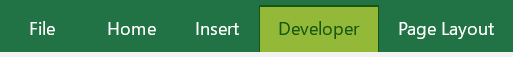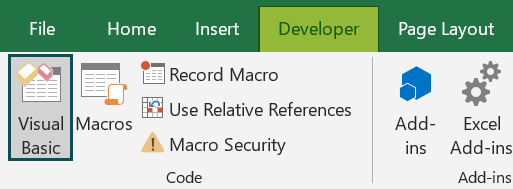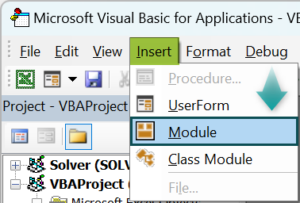2. Initialize a sub-procedure to count a given word’s occurrences in a table.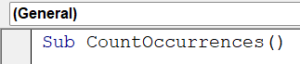The table in question is shown below: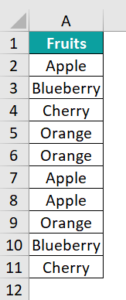3. Declare the range, the count variable, the value to be searched, and the Worksheet we’ll be working on.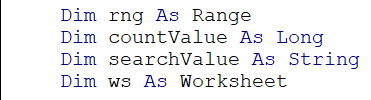4. Set the worksheet on which this subroutine will be working.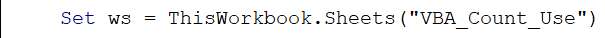5. Set the range of cells for the subroutine.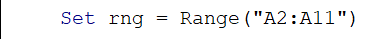6. Declare the value in the above table to be searched.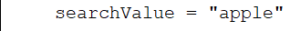7. Count the occurrences of the word “apple” in the table and print the number of times the word “apple” has been repeated.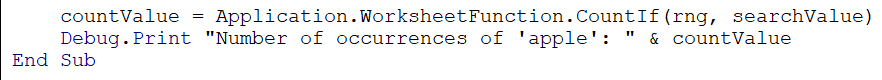Since the COUNTIF function is also a part of the “WorksheetFunction” object in Excel VBA, while calling COUNT or COUNTIF function, we call it with the prefix “Application.WorksheetFunction.” Now print the count value for the word “apple.”

Code:

Sub CountOccurrences()
Dim rng As Range
Dim countValue As Long
Dim searchValue As String
Dim ws As Worksheet
Set ws = ThisWorkbook.Sheets(“VBA_Count_Use”)
Set rng = Range(“A2:A11”)
searchValue = “apple”
countValue = Application.WorksheetFunction.CountIf(rng, searchValue)
Debug.Print “Number of occurrences of ‘apple’: ” & countValue
End Sub

8. Using “F5,” run the code and see the output printed in the Immediate tab.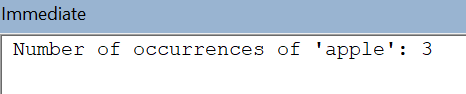### Examples

Let us look at a few examples to see how VBA Count can be used in VBA code.

#### Example #1

Consider an example where we need to display the distinct salesperson present in the table using VBA Count rows.

• Step 1: Define a sub-procedure that finds distinct values for the “Sales Rep” column in column B.
• Step 2: Using the Rows, we perform VBA Count Rows for the above Table.count function and find the last non-empty row using the xlUp or XLOOKUP function. Here, we’re taking a random column and counting the number of rows since all the columns will have the same number of rows in a table.
• Step 3: To find the distinct values in the Sales Rep column, we use the AdvancedFilter method to filter unique values.

First, declare the range of the filter method; here, it starts from cell B2 till the last row of the column, which we’ve found earlier. To that range, perform VBA AdvancedFilter, and copy the filtered values using xlFilterCopy in “Action,” then copy those values to column “E.” Set Unique as “True” so that only the distinct values are added.

Code:

Sub CountUniqueSalesReps()
Dim row As Long
row = Cells(Rows.count, “C”).End(xlUp).row
Action:=xlFilterCopy, CopyToRange:=ActiveSheet.Range(“E2”), _
Unique:=True
End Sub

• Step 5: Run the code to print the distinct sales representatives in the table.

#### Example #2

Consider an example where you need to count the number of students who have scored above 80 in their exams, as seen in the table below:

• Step 1: Initialize a subroutine to count the number of students who scored above 80.
• Step 2: Declare a variable to store the worksheet in which this subroutine will work. The range of the table is defined using the Range variable in Excel VBA. The threshold marks will be double if the given input is in decimal format, and then the count value to store the number of rows with values above 80.
• Step 3: Declare the worksheet in which this subroutine will work.
• Step 4: To find the number of values above 80, we declare the range of the scores only for the Count function to work effectively.
• Step 5: Set the threshold value.
• Step 6: Using the COUNTIF function, find the count of rows with scores above 80 and print the number of rows.

Code:

Sub countAboveThreshold()
Dim ws As Worksheet
Dim scoreRange As Range
Dim threshold As Double
Dim countAboveThreshold As Long
Set ws = ThisWorkbook.Worksheets(“Example_2”)
Set scoreRange = ws.Range(“B2:B20”)
threshold = 80
countAboveThreshold = Application.WorksheetFunction.CountIf(scoreRange, “> ” & threshold)
Debug.Print “Number of students with scores above ” & threshold & “: ” & countAboveThreshold
End Sub

• Step 7: Print the count of the number of rows above the threshold value by running the code above using “F5” or clicking the “Run” button in the VBA toolbar. The output is shown below.

### Important Things to Note

• Do specify the range or collection: Provide a valid range or collection as the argument for the Count function. This ensures the accurate counting of elements.
• Do use the Application.WorksheetFunction.Count syntax: Since Count is a worksheet function, it is recommended to use it with the Application.WorksheetFunction prefix to access the function in VBA.
• Do assign the result to a variable: Capture the result of the Count function in a variable for further processing or displaying.
• Don’t mix up Count and CountA: The Count function counts the number of numeric values in a range, while CountA counts the number of non-empty cells (including text, numbers, and logical values). Ensure you use the appropriate function based on your requirements.
• Don’t use Count for non-numeric values: The Count function only counts numeric values in a range. If you want to count non-numeric values or cells meeting specific criteria, consider using other functions like CountIf or specialized collection objects.
• Don’t forget to include the necessary library references: If you’re using early binding and writing code in a VBA module, make sure to include the appropriate library reference (e.g., Microsoft Excel Object Library) to access the Count function.

1. Why is VBA Count function not working?

• Invalid range or collection
• Syntax error: Check for any syntax errors in your code.
• Incorrect library reference: Include the necessary library reference (e.g., Microsoft Excel Object Library).
• Non-numeric values in the range: The Count function counts numeric values only.
• Error handling: If there are potential errors in your code, implement error handling techniques to handle them gracefully.

2. How do I count cells in a column in VBA?

To perform VBA count columns:
Define a range object that represents the column you want to count.
Use the WorksheetFunction.CountA function along with the range object to count non-empty cells in the column.
Assign the count value to a variable for further use or display.

3. How to VBA Count specific characters in a string?

Use the LEN function to get the length of the string.
Loop through each character in the string using a For loop.
Check if the current character matches the specific character you want to count.
Increment a counter variable each time a match is found.
The final value of the counter variable will represent the count of specific characters in the string.

4. How to VBA Count files in folder?

Yes, it is possible to replace a string in Excel VBA without using the Replace function by following these steps:
Use the FileSystemObject to access the file system.
Create a FileSystemObject variable using the CreateObject function.
Use the GetFolder method of the FileSystemObject to get a reference to the folder.
Use the folder object’s Files property to access the file collection.
Use the Count property of the files collection to get the count of files.